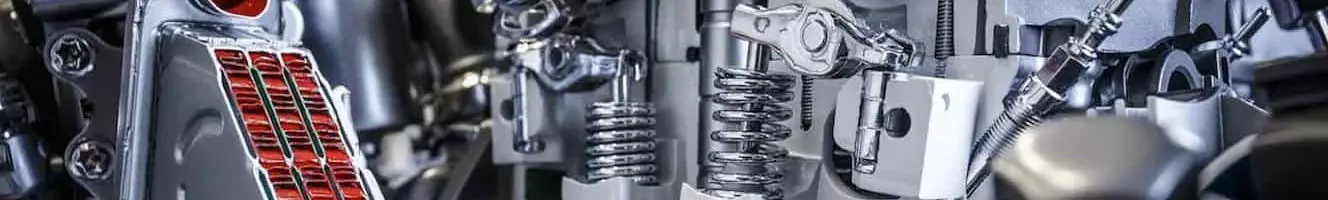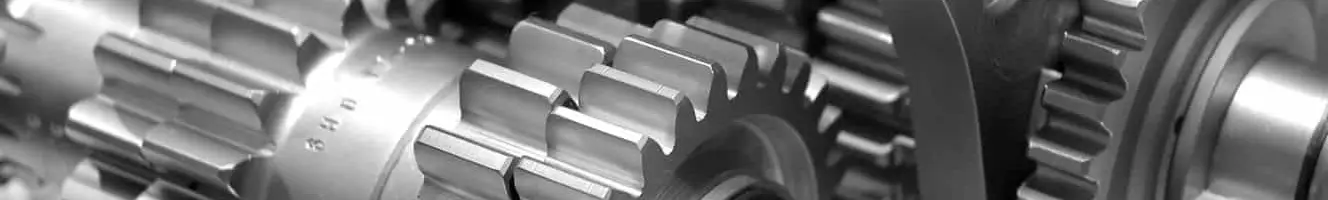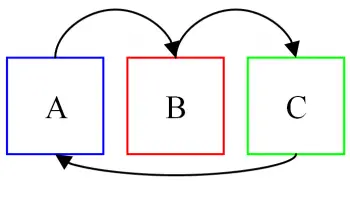Diesel engine. Diesel cycleEndothermic engineGearbox

# Zeroth law of thermodynamicsThe zeroth law of thermodynamics states that "if two bodies A and B are separately in thermal equilibrium with a third body C, then A and B are in thermal equilibrium with each other.

This law allows the definition of a temperature range, such as temperature scales Celsius, Fahrenheit, Kelvin, Réaumur, Rankine, Newton and Leiden.

## Statement of the zeroth law of thermodynamics

The enunciation of the zeroth law of thermodynamics is defined as: Two systems in thermal equilibrium with a third are in equilibrium with each other.

The zeroth law of thermodynamics allows us to differentiate bodies from each other with respect to their "degree of warming". This attribute, which is a property of the system, we will identify with its temperature, which turns out to be a macroscopic (measurable) concept.

Through these concepts we can understand the functioning of the devices called thermometers, which are the devices that precisely measure the temperature property of bodies.

It should be noted that the formulation of the zeroth law of thermodynamics contains three firm ideas:

• The existence of a state variable, called temperature.
• The equality of temperatures as a condition for thermal equilibrium between two systems, or between parts of the same system.
• The existence of a relationship between the independent variables of the system and the temperature, called the equation of state.

## Temperature measurement

The intuitive concept we have about temperature is very subjective. The words hot and cold were created to facilitate the understanding of the thermal sensation. This feeling varies for each person, so it is not considered. These terms serve to help us understand the theory.

For example, if on a cold day we touch a wooden object and a metal object, both at room temperature, for example, we will have the impression that the metal object is colder. This happens because, as our body is not in thermal equilibrium with them, there will be energy exchange in the form of heat between our body and objects. Since metal is a better conductor than wood, heat will be transferred more quickly and it is that rapid change of energy that gives us the impression that the material is colder. Therefore, instead of these notions.

When a body is heated or cooled, certain characteristics can be modified such as volume, length or electrical resistance. The variation of these characteristics can be related to its temperature variation and from that it is possible to create an instrument that measures the temperature on a certain scale.

valoración: 2 - votos 2

Last review: April 26, 2018